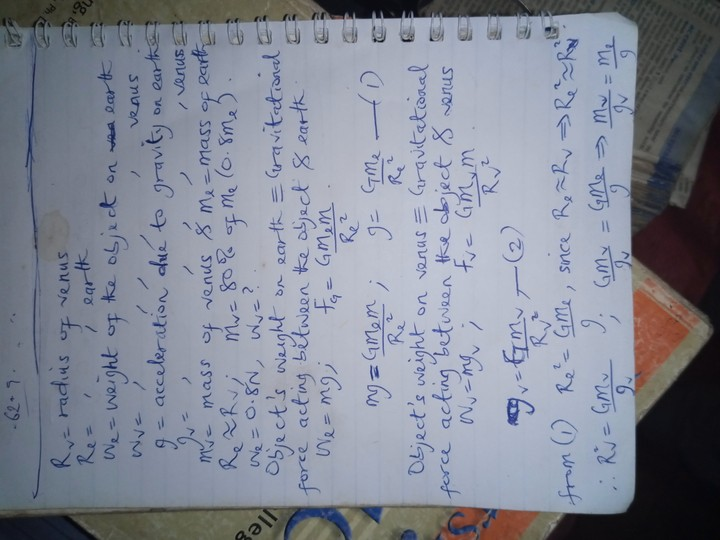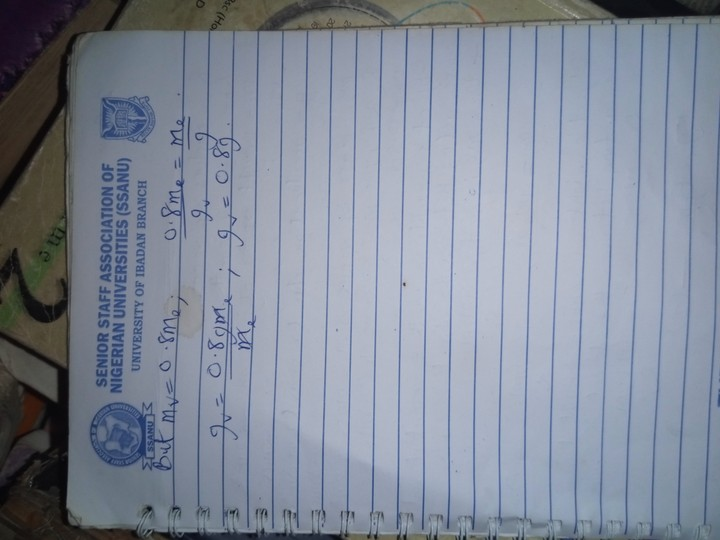#Welcome, Guest: Register On Nairaland / LOGIN! / Trending / Recent / New
Stats: 3,037,064 members, 7,448,811 topics. Date: Friday, 02 June 2023 at 11:30 PM

## Physics Question - Education - Nairaland

Nairaland Forum / Nairaland / General / Education / Physics Question (1449 Views)

 Physics Question by blendz4real(m): 9:39pm On Aug 01, 2019 Some one pls help answer urgently!The radius of the planet Venus is nearly thesame as that of the earth but it's mass is only 80% that of the earth. If an object weighs 0.8 on the earth what does it weigh on Venus?Calculate the value of g on venus.Thanks Re: Physics Question by Nobody: 5:19pm On Aug 03, 2019 I hope you can understand itRe: Physics Question by Nobody: 5:22pm On Aug 03, 2019 Concluding solutionRe: Physics Question by Martinez39(m): 8:50pm On Aug 03, 2019 blendz4real:Some one pls help answer urgently!The radius of the planet Venus is nearly thesame as that of the earth but it's mass is only 80% that of the earth. If an object weighs 0.8 on the earth what does it weigh on Venus?Calculate the value of g on venus.Thanks Since the radius of Venus, Rv , and the radius of Earth, Re , are nearly the same(ie. approximately equal) and no further information is given on their relative sizes, we assume thatRv = Re = RBy Newton's law of gravitation, we have the following On a planet of mass, M , and radius, r , the weight, W, of an object of mass, m , is given by W = (GMm)/r² = mg Where:g = the acceleration due to gravity on the planet, andG = the gravitational constant From the question, the mass of Venus, Mv , and the mass of Earth, Me are given by Mv = (4/5)Me The weights of the object on Venus and Earth,Wv & We = 0.8 respectively, are given by Wv = (GMvm)/R² = [(4/5)(GMem)]/R² = mgv ———(1) We = (GMem)/R² = mge ———(2)ge = 9.8 m/s²gv =? Equations (1) & (2) can be rewritten as follows (5/4)Wv = We = (GMem)/R²——and—— m = Wv/gv = We/ge (5/4)Wv = 0.8 Wv = (4/5) × 0.8 = 0.640.8/9.8 = 0.64/gvgv = (0.64 × 9.8 )/0.8 = 7.84 m/s² 1 Like Re: Physics Question by Nobody: 9:14pm On Aug 03, 2019 .. Re: Physics Question by blendz4real(m): 9:11pm On Aug 08, 2019 gensteejay:Concluding solution Thanks for your input. Re: Physics Question by blendz4real(m): 9:12pm On Aug 08, 2019 Martinez39: Since the radius of Venus, Rv , and the radius of Earth, Re , are nearly the same(ie. approximately equal) and no further information is given on their relative sizes, we assume thatBy Newton's law of gravitation, we have the following From the question, the mass of Venus, Mv , and the mass of Earth, Me are given by The weights of the object on Venus and Earth,Wv & We = 0.8 respectively, are given by Equations (1) & (2) can be rewritten as follows (5/4)Wv = 0.8[/i] [/font]Wv = (4/5) × 0.8 = 0.640.8/9.8 = 0.64/gvgv = (0.64 × 9.8 )/0.8 = 7.84 m/s² Thanks alot.... I appreciate